Using Loop in VBA in Microsoft Excel

In this article, we have covered different kinds of loops used in VBA and how to use them to accomplish the same task in different ways.

Why Loops?

Looping is one of the most powerful programming techniques used across many programming languages. Looping is used to repeat a block of code for required number of times or until a given condition evaluates to true or a specific value is reached, after which the next block of code is executed.

The purpose of an Excel VBA loop is to make Excel repeat a piece of code certain number of times. One can specify how many times a code must be repeated as a fixed number (e.g. do this 10 times), or as a variable (e.g. do this as many times as there are rows of data).

Excel Loops can be constructed in different ways to suit different circumstances. Often, same results can be obtained in different ways to suit your personal preferences.

There are three different kinds of loops available in Excel VBA, which are:

1. DO UNTIL Loop

2. DO WHILE Loop

3. FOR Loop

1. DO UNTIL Loop

The DO UNTIL Loop is used to repeat a block of code indefinitely, until the specified condition is set to True. The condition can either be checked at the beginning or at the end of the Loop. The DO UNTIL … LOOP statement tests the condition at the beginning, whereas the DO … LOOP UNTIL statement tests the condition at the end of the Loop.

Syntax of DO UNTIL … LOOP statement

Do Until [Condition]

[Block of code to be repeated]

Loop

Syntax of DO … LOOP UNTIL statement

Do

[Block of code to be repeated]

Loop Until [Condition]

We have explained DO… UNTIL loop with an example. Loop1 and Loop2 macros are used to calculate the average of numbers in column A and column B using the DO… UNTIL loop.

Sample data is present in the range A15:B27. Column A contains scores of Round 1 and column B contains scores of Round 2. We want to calculate averages of scores in Round 1 and Round 2 in column C.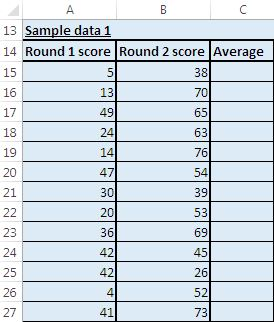In Loop1 macro, we have used “FormulaR1C1” to insert average formula in the active cell. Condition statement in the DO UNTIL loop is checked at the end of the loop.

In Loop2 macro, we have used “WorksheetFunction.Average” to insert average value in the active cell. Even in this macro, condition statement is checked at the end of the loop.

The only difference between Loop1 and Loop2 macro is that Loop1 inserts the average formula, whereas Loop2 calculates the average and then inserts the average value in the active cell.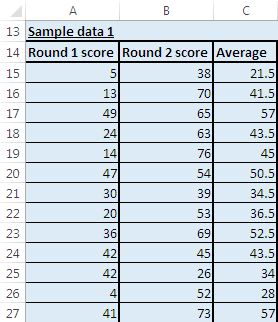2. DO WHILE Loop

The DO WHILE Loop is used to repeat a block of code indefinite number of times, while the specified condition continues to be True and stops when the condition returns False. The condition can either be checked in the beginning or at the end of the Loop. The DO WHILE … LOOP statement tests the condition in the beginning, whereas the DO … LOOP WHILE statement tests the condition at the end of the loop. The DO … LOOP WHILE statement is used when we want the loop to run the block of code at least once before checking for the condition.

Syntax of DO WHILE … LOOP statement

Do While [Condition]

[Block of code to be repeated]

Loop

Syntax of DO … LOOP WHILE statement

Do

[Block of code to be repeated]

Loop While [Condition]

In this example, Loop3 and Loop4 macros are used to calculate averages for values in cells of column A and column B. Both macros work on the same sample data as used by macros Loop1 and Loop2. Both use DO WHILE statement to loop through the range which contains the data.

The only difference between Loop3 and Loop4 macros is that they are different ways of representing conditions of DO WHILE loop.

As Loop3 and Loop4 macros use same input data and even perform same functions as Loop1 macro, so that the output returned will also be same as of Loop1 macro.

3. FOR loop

The For Loop is used to repeat a block of code for specific number of times.

Syntax of FOR loop

For count_variable = start_value To end_value

[block of code]

Next count_variable

Loop5 macro shows how to use FOR loop to calculate the average. It also uses the same sample data used by other macros. We have used 15 as starting value as the sample data starts from the 15th row. We have used Range(“A” & Cells.Rows.Count).End(xlUp).Row to find the last row containing data. FOR loop will repeat (lastcell- 15) number of times.

Output returned after running Loop5 macro is same as of Loop1 macro.

Loop6 macro is created to calculate average, only if the active cell which will have the average function is empty before running the macro.

Sample data for this macro is present in the range E15 to G27.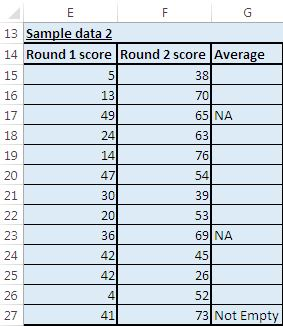We have used DO… LOOP WHILE to loop through the defined range. IF statement is used to check whether the cell where function will be inserted, contains a value. This macro will insert average function to the cell only if it is empty.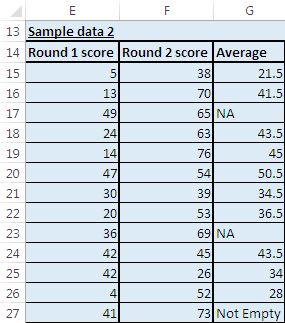Loop7 macro is also used to calculate average. It checks for values in the helper column before evaluating whether to loop again. It also checks whether the cell reference to be used in the average function is empty.

Sample data used for Loop7 macro is in the range J15:M27.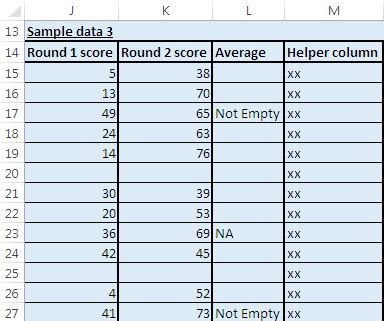Column M is used as helper column. This macro will insert an average function only if a cell in column M is non empty. This macro checks that a cell should be empty before inserting an average function in it. It will not insert an average function if the cell referenced in the average function is empty.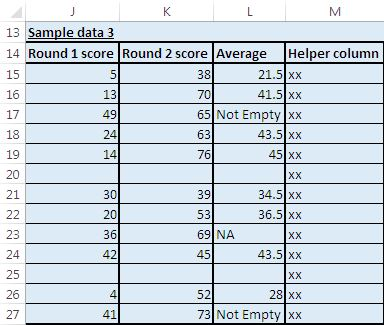```
Option Explicit

Sub Loop1()

'Calculating average
'Do Until loop will loop until cell in the previous column of active cell is empty

Range("C15").Select

Do
'Assigning average function on value in cells of previous two consecutive columns
ActiveCell.FormulaR1C1 = "=Average(RC[-1],RC[-2])"

'Moving to cell in next row
ActiveCell.Offset(1, 0).Select

'Checking whether value in cell of previous column is empty
'Do Until loop will loop until condition statement returns True
Loop Until IsEmpty(ActiveCell.Offset(0, -1))

Range("A15").Select

End Sub

Sub Loop2()

'Calculating average
'Do Until loop will loop until cell in the previous column of active cell is empty
'This macro is similar to macro Loop1, only way of calculating average is different

Range("C15").Select

Do
'Worsheet.Average function is used for calculating the average
ActiveCell.Value = WorksheetFunction.Average(ActiveCell.Offset(0, -1).Value, _
ActiveCell.Offset(0, -2).Value)

ActiveCell.Offset(1, 0).Select
Loop Until IsEmpty(ActiveCell.Offset(0, -1))

Range("A15").Select

End Sub

Sub Loop3()

'Calculating average
'Do While loop will run until cell in the previous column of active cell is empty

Range("C15").Select

'Checking whether value in cell of previous column is empty
'Do While loop will loop until condition statement is True
Do While IsEmpty(ActiveCell.Offset(0, -1)) = False

'Assigning average function on value in cells of previous two consecutive column
ActiveCell.FormulaR1C1 = "=Average(RC[-1],RC[-2])"

'Moving to cell in next row
ActiveCell.Offset(1, 0).Select
Loop

Range("A15").Select

End Sub

Sub Loop4()

'Calculating average
'Do While loop will run until cell in the previous column of active cell is empty
'This macro is similar to macro Loop3, only way of applying condition is different

Range("C15").Select

Do While Not IsEmpty(ActiveCell.Offset(0, -1))
ActiveCell.FormulaR1C1 = "=Average(RC[-1],RC[-2])"
ActiveCell.Offset(1, 0).Select
Loop

Range("A15").Select

End Sub

Sub Loop5()

'FOR loop repeats for a fixed number of times determined by the number of rows

Dim i, lastcell As Long

'Finding the last row containing data in column A
lastcell = Range("A" & Cells.Rows.Count).End(xlUp).Row

Range("C15").Select

'i variable is assigned value of 15 as our sample data begin from 15th row
'FOR Loop will loop x
For i = 15 To lastcell
ActiveCell.FormulaR1C1 = "=Average(RC[-1],RC[-2])"
ActiveCell.Offset(1, 0).Select
Next i

Range("A15").Select

End Sub

Sub Loop6()

'Calculating average
'Do Until loop will loop until cell in the previous column of active cell is empty
'It does not calculate an average if there is already something in the cell

Range("G15").Select

Do
If IsEmpty(ActiveCell) Then
ActiveCell.FormulaR1C1 = "=Average(RC[-1],RC[-2])"
End If

ActiveCell.Offset(1, 0).Select
Loop Until IsEmpty(ActiveCell.Offset(0, -1))

Range("E15").Select

End Sub

Sub Loop7()

'Do Until loop runs as long as there is something in cell in next column
'It does not calculate an average if there is already something in the active cell
'Nor if there is no data in cells which are used within average function (to avoid #DIV/0 errors).

'Calculating average

Range("L15").Select

Do
If IsEmpty(ActiveCell) Then
If IsEmpty(ActiveCell.Offset(0, -1)) And IsEmpty(ActiveCell.Offset(0, -2)) Then
ActiveCell.Value = ""
Else
ActiveCell.FormulaR1C1 = "=Average(RC[-1],RC[-2])"
End If
End If

ActiveCell.Offset(1, 0).Select
Loop Until IsEmpty(ActiveCell.Offset(0, 1))

Range("J15").Select

End Sub

```

If you liked this blog, share it with your friends on Facebook. Also, you can follow us on Twitter and Facebook.

We would love to hear from you, do let us know how we can improve our work and make it better for you. Write to us at info@exceltip.com

## Users are saying about us...

1.Thanks for the tip! I have a worksheet with a column that has intermittent empty cells. I have a macro that populates all of the empty cells below a populated cell with that cell’s value. I applied the Loop(2) technique and it continued on and kept going until I “ESC” out. I’m wondering if you can tell me what I did incorrectly? Thanks again!

2.Marcelo Liascovich

Thanks you
It’s very useful

Which is the best way to introduce to Excel VBA. I am 46 year old and I think happy to learn about excel

3.Greatly simplified looping options.

4.This page gives an excellent overview of different loops and their syntax.I need still more on loops and their usage examples.

5.The following can be used to delete unwanted lines from a large sheet .

6.“The following can be used to delete unwanted lines from a large sheet .

Do
if activecell.value=”””” then
selection.entirerow.delete
else
dim row
row=1
selection.offset(row).select
end if
loop until activecell.value=””Target”” “

7.“Why bother with this when you can just use an If Statement…
=IF(A2=0,0,A1/A2) Surely, there must be a better way?”

8.If I talk about exercise 5 the given code is not working properly. After running the macros It is giving result for C1 only.
A B C
6 7 6.5
16 18
17 6
18 9
15 6
16 17

The code is:
Sub Loop3()
‘ This loop runs as long as there is something in the NEXT column
‘ It does not calculate an average if there is already something in the cell
‘ nor if there is no data to average (to avoid #DIV/0 errors).
Do
If IsEmpty(ActiveCell) Then
If IsEmpty(ActiveCell.Offset(0, -1)) And IsEmpty(ActiveCell.Offset(0, -2)) Then
ActiveCell.Value = “”
Else
ActiveCell.FormulaR1C1 = “=Average(RC[-1],RC[-2])”
End If
End If
ActiveCell.Offset(1, 0).Select
Loop Until IsEmpty(ActiveCell.Offset(0, 1))
End Sub

9.Pls ignore the last one:

If I talk about exercise 5 the given code is not working properly. After running the macros It is giving result for C1 only.
A B C
6 7 6.5
16 18
17 6
18 9
15 6
16 17

The code is:
Sub Loop3()
‘ This loop runs as long as there is something in the NEXT column
‘ It does not calculate an average if there is already something in the cell
‘ nor if there is no data to average (to avoid #DIV/0 errors).
Do
If IsEmpty(ActiveCell) Then
If IsEmpty(ActiveCell.Offset(0, -1)) And IsEmpty(ActiveCell.Offset(0, -2)) Then
ActiveCell.Value = “”
Else
ActiveCell.FormulaR1C1 = “=Average(RC[-1],RC[-2])”
End If
End If
ActiveCell.Offset(1, 0).Select
Loop Until IsEmpty(ActiveCell.Offset(0, 1))
End Sub
Please suggest whats going wrong with me.

10.How do I do this without using active cell (i.e: starting with a particular cell)

11.I HAVE SAVED EXCEL FILE TODAY… I WANT TO RECOVER THE SAME EXCEL FILE HAVING DATA OF YESTERDAYS.

12.Thanks for this – very useful introduction to loops

Can I use a loop function to expand a column of cells so that each cell is repeated on 4 rows, i.e the value of A1 appears in B1,B2,B3,B4
the value of A2 appears in B5,B6,B7,B8
etc.

Can I also do this but introduce 3 rows of empty cells between each exisiting cell?

Thanks!

13.I want to Replace some words from my data with specific words which i have in another excel sheet..
Is there any way to do so..
For example- I have some addresses in a sheet and in another sheet i have some words and their short form..now i want to put these short forms in my addresses from another sheet…

14.Very simplified looping options, anyway very good thanks a lot.

15.How can I write the loop for VBA?

16.I am 80 years old male and trying to prevent debenture, hence taking up excel,but would be happy to get any information at all.
I have started by working out my Gas bill. the main problem i am having is try to transfer data from one point to another.
I have set it out to produce cost Daily , Weekly and Yearly, i would like to transfer that data to a table sort of; using one row across four columns including the date, all from the calculations filling the columns row by row.
Sorry i may seem simple compared to you Excel cleverer people, but I am just learning the basics.
thanks a lot

•Hi John,

Thanks for writing us.

We would be glad to help you if you can give more clarity on your requirement and can share a sample data with us. Also, to get an instant solution, you can login at http://www.excelforum.com and can ask simple or complicated queries to our expert.

Happy Learning

17.SerenaMikell

Thanks you for sharing one of the most powerful programming techniques.

18.You marked your post perfect with your great writing skill, thanks for that. I need to create a floating summary report in excel sheet. I also try here http://www.excelforum.com/showthread.php?t=1139964 but till now no result found.

19.20.